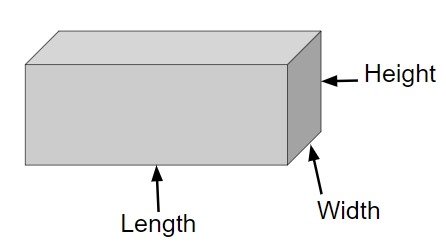# Program for Volume and Surface Area of Cuboid in C++

## What is cuboid?

Cuboid is a three-dimensional object with six faces of rectangle shape which means it has sides of different length and breadth. The difference between a cube and cuboid is that a cube has equal length, height and breadth whereas in cuboids these three are not same

Properties of cuboid are −

• six faces
• 12 edges
• 8 vertices

Given below is the figure of cube## Problem

Given with the length, width and volume, the task is to find the total surface area and volume of a cuboid where surface area is the space occupied by the faces and volume is the space that a shape can contain.

To calculate surface area and volume of a cuboid there is a formula

Surface Area = 2(|*w + w * h + |*h )

Volume = L* W * H

## Example

Input-: L=3 H=2 W=3
Output-: Volume of cuboid is: 18
Total Surface Area of cuboid is: 42

## Algorithm

Start
Step 1 -> declare function to find volume of cuboid
double volume(double l, double h, double w)
return (l*h*w)
Step 2 -> declare function to find area of cuboid
double surface_area(double l, double h, double w)
return (2 * l * w + 2 * w * h + 2 * l * h)
Step 3 -> In main()
Declare variable double l=3, h=2 and w=3
Print volume(l,h,w)
Print surface_area(l, h ,w)
Stop

## Example

#include <bits/stdc++.h>
using namespace std;
//function for volume of cuboid
double volume(double l, double h, double w){
return (l * h * w);
}
//function for total surface area of cuboid
double surface_area(double l, double h, double w){
return (2 * l * w + 2 * w * h + 2 * l * h);
}
int main(){
double l = 3;
double h = 2;
double w = 3;
cout << "Volume of cuboid is: " <<volume(l, h, w) << endl;
cout << "Total Surface Area of cuboid is: "<< surface_area(l, h, w);
return 0;
}

## Output

Volume of cuboid is: 18
Total Surface Area of cuboid is: 42